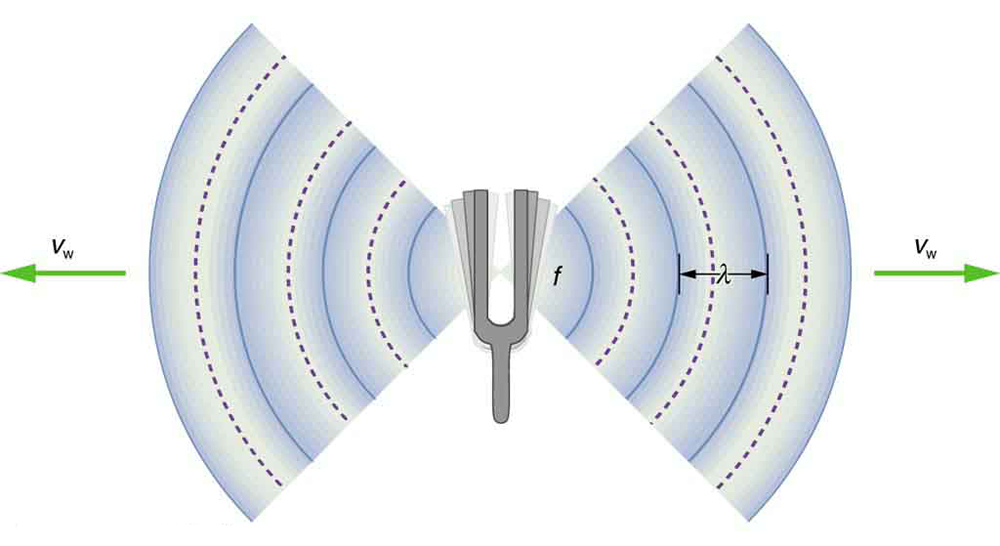Physics

# Multiple Choice

PhysicsMultiple Choice

### Multiple Choice

#### 14.1Speed of Sound, Frequency, and Wavelength

34.

What properties does a loud, shrill whistle have?

1. high amplitude, high frequency
2. high amplitude, low frequency
3. low amplitude, high frequency
4. low amplitude, low frequency
35.
What is the speed of sound in fresh water at 20 degrees Celsius?
1. 5960\,\text{m}/\text{s}
2. 1540\,\text{m}/\text{s}
3. 331\,\text{m}/\text{s}
4. 1480\,\text{m}/\text{s}
36.A tuning fork oscillates at a frequency of 512 Hz. If sound is traveling at 345 m/s, how many wave peaks will reach the eardrum of a person sitting near that fork in 2 seconds?
3. Slightly over 500.
4. Slightly over 1,000.
37.
Why does the amplitude of a sound wave decrease with distance from its source?
1. The amplitude of a sound wave decreases with distance from its source, because the frequency of the sound wave decreases.
2. The amplitude of a sound wave decreases with distance from its source, because the speed of the sound wave decreases.
3. The amplitude of a sound wave decreases with distance from its source, because the wavelength of the sound wave increases.
4. The amplitude of a sound wave decreases with distance from its source, because the energy of the wave is spread over a larger and larger area.
38.
Does the elasticity of the medium affect the speed of sound? If so, how?
1. No, there is no relationship that exists between the speed of sound and elasticity of the medium.
2. Yes. When particles are more easily compressed in a medium, sound does not travel as quickly through the medium.
3. Yes. When the particles in a medium do not compress much, sound does not travel as quickly through the medium.
4. No, the elasticity of a medium affects frequency and wavelength, not wave speed.

#### 14.2Sound Intensity and Sound Level

39.

Which of the following terms is a useful quantity to describe the loudness of a sound?

1. intensity
2. frequency
3. pitch
4. wavelength
40.

What is the unit of sound intensity level?

1. decibels
2. hertz
3. watts
41.
If a particular sound S1 is 5 times more intense than another sound S2, then what is the difference in sound intensity levels in dB for these two sounds?
1. 5\,\text{dB}
2. 6\,\text{dB}
3. 7\,\text{dB}
42.
By what minimum amount should frequencies vary for humans to be able to distinguish two separate sounds?
1. 100\,\text{Hz}
2. 10\,\text{Hz}
3. 5\,\text{Hz}
4. 1\,\text{Hz}
43.

Why is I0 chosen as the reference for sound intensity?

1. Because, it is the highest intensity of sound a person with normal hearing can perceive at a frequency of 100 Hz.
2. Because, it is the lowest intensity of sound a person with normal hearing can perceive at a frequency of 100 Hz.
3. Because, it is the highest intensity of sound a person with normal hearing can perceive at a frequency of 1000 Hz.
4. Because, it is the lowest intensity of sound a person with normal hearing can perceive at a frequency of 1000 Hz.

#### 14.3Doppler Effect and Sonic Booms

44.
In which of the following situations is the Doppler effect absent?
1. The source and the observer are moving towards each other.
2. The observer is moving toward the source.
3. The source is moving away from the observer.
4. Neither the source nor the observer is moving relative to one another.
45.
What does the occurrence of the sonic boom depend on?
1. speed of the source
2. frequency of source
3. amplitude of source
4. distance of observer from the source
46.
What is the observed frequency when the observer is moving away from the source at 125\,\text{m/s}? The source frequency is 237\,\text{Hz} and the speed of sound is 325\,\text{m/s}.
1. 303\,\text{Hz}
2. 259\,\text{Hz}
3. 201\,\text{Hz}
4. 146\,\text{Hz}
47.
How will your perceived frequency change if the source is moving towards you?
1. The frequency will become lower.
2. The frequency will become higher.

#### 14.4Sound Interference and Resonance

48.

Observation of which phenomenon can be considered proof that something is a wave?

1. interference
2. noise
3. reflection
4. conduction
49.

Which of the resonant frequencies has the greatest amplitude?

1. The first harmonic
2. The second harmonic
3. The first overtone
4. The second overtone
50.
What is the fundamental frequency of an open-pipe resonator?
1. 3\,\text{v}/2\,\text{L}
2. 2\,\text{v}/\text{L}
3. \text{v}/\text{L}
4. \text{v}/2\,\text{L}
51.
What is the beat frequency produced by the superposition of two waves with frequencies 300\,\text{Hz} and 340\,\text{Hz}?
1. 640\,\text{Hz}
2. 320\,\text{Hz}
3. 20\,\text{Hz}
4. 40\,\text{Hz}
Order a print copy

As an Amazon Associate we earn from qualifying purchases.